# 逻辑斯谛回归与最大熵模型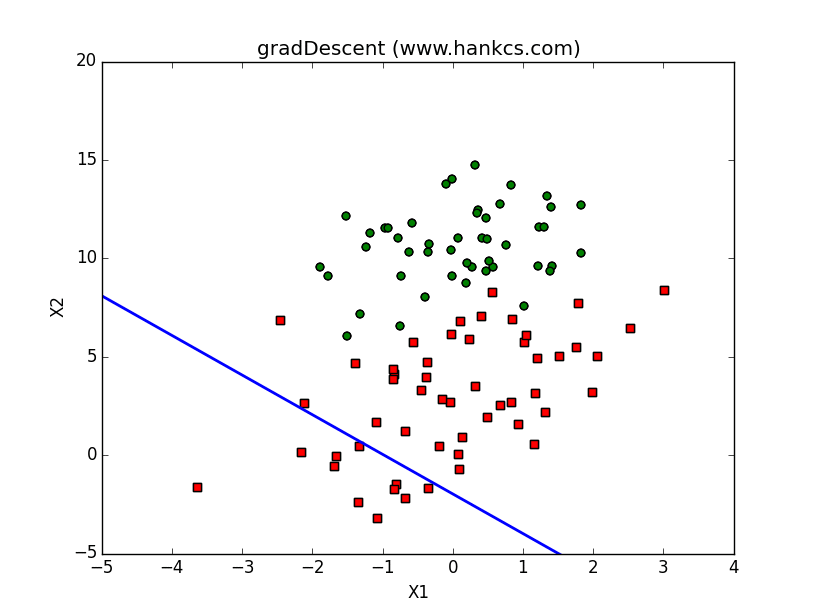本文希望通过《统计学习方法》 第六章的学习，由表及里地系统学习最大熵模型。文中使用Python实现了逻辑斯谛回归模型的3种梯度下降最优化算法，并制作了可视化动画。针对最大熵，提供一份简明的GIS最优化算法实现，并注解了一个IIS最优化算法的Java实现。

## 逻辑斯谛回归模型

### 逻辑斯谛分布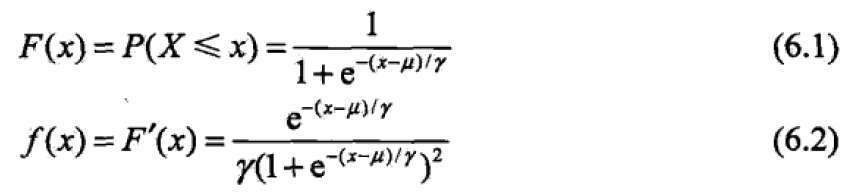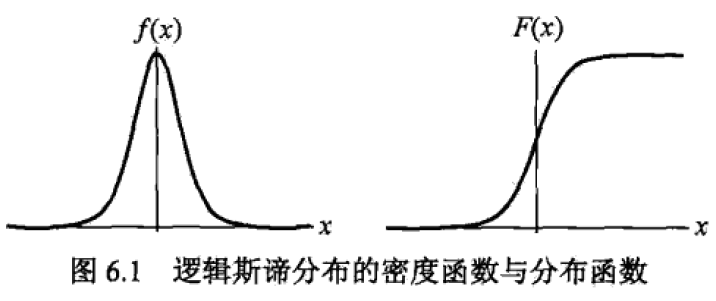$$F(u-x)-\frac{1}{2}=-F(u+x)+\frac{1}{2}$$

### 二项逻辑斯蒂回归模型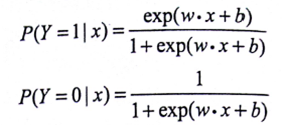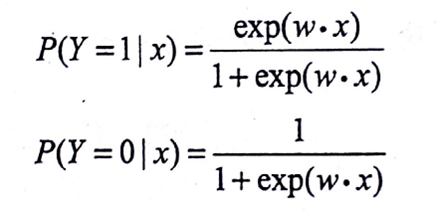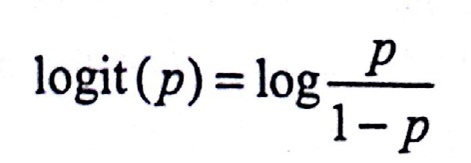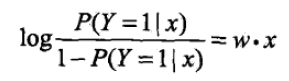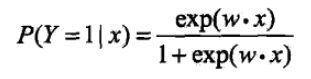### 模型参数估计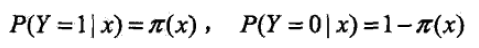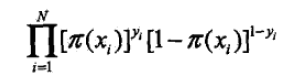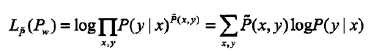## 逻辑斯谛回归实现

### 测试数据

《机器学习实战》中给出了一个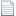testSet.txt

-0.017612	14.053064	0
-1.395634	4.662541	1
-0.752157	6.538620	0
-1.322371	7.152853	0
0.423363	11.054677	0
0.406704	7.067335	1
0.667394	12.741452	0
-2.460150	6.866805	1
0.569411	9.548755	0
-0.026632	10.427743	0

def loadDataSet():
"""
加载数据集

:return:输入向量矩阵和输出向量
"""
dataMat = []; labelMat = []
fr = open('testSet.txt')
lineArr = line.strip().split()
dataMat.append([1.0, float(lineArr), float(lineArr)]) #X0设为1.0，构成拓充后的输入向量
labelMat.append(int(lineArr))
return dataMat,labelMat

def plotBestFit(weights):
"""
画出数据集和逻辑斯谛最佳回归直线
:param weights:
"""
import matplotlib.pyplot as plt
dataArr = array(dataMat)
n = shape(dataArr)
xcord1 = []; ycord1 = []
xcord2 = []; ycord2 = []
for i in range(n):
if int(labelMat[i])== 1:
xcord1.append(dataArr[i,1]); ycord1.append(dataArr[i,2])
else:
xcord2.append(dataArr[i,1]); ycord2.append(dataArr[i,2])
fig = plt.figure()
ax.scatter(xcord1, ycord1, s=30, c='red', marker='s')
ax.scatter(xcord2, ycord2, s=30, c='green')
if weights is not None:
x = arange(-3.0, 3.0, 0.1)
y = (-weights-weights*x)/weights   #令w0*x0 + w1*x1 + w2*x2 = 0，其中x0=1，解出x1和x2的关系
ax.plot(x, y)                               #一个作为X一个作为Y，画出直线
plt.xlabel('X1'); plt.ylabel('X2');
plt.show()

plotBestFit(None)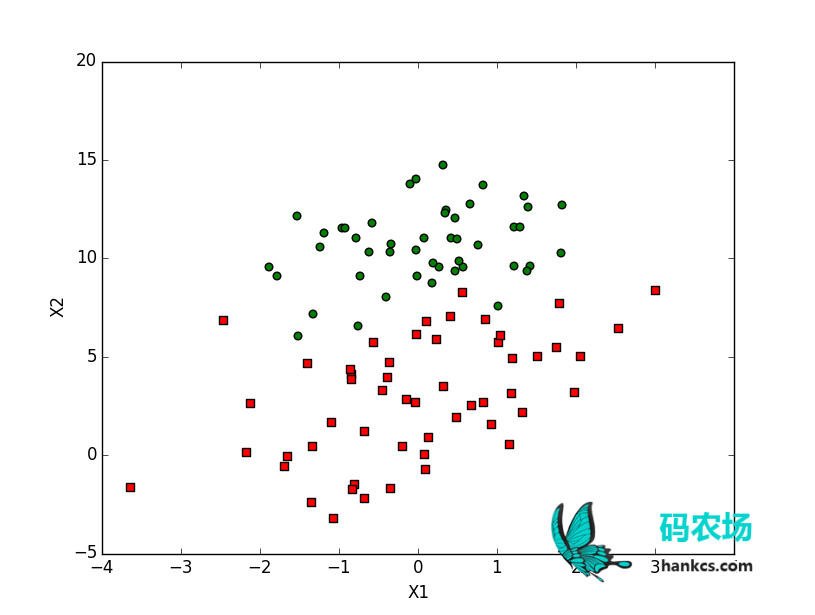### 梯度下降算法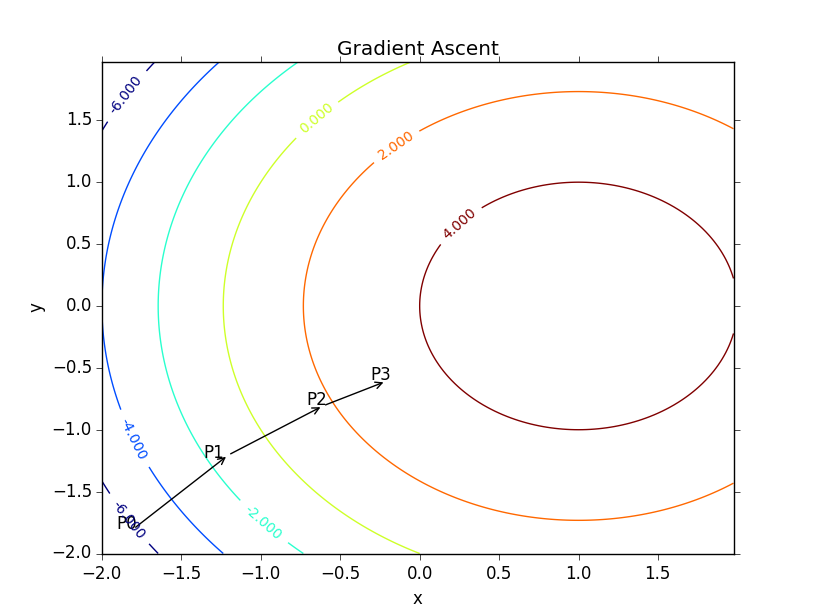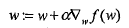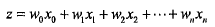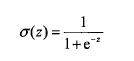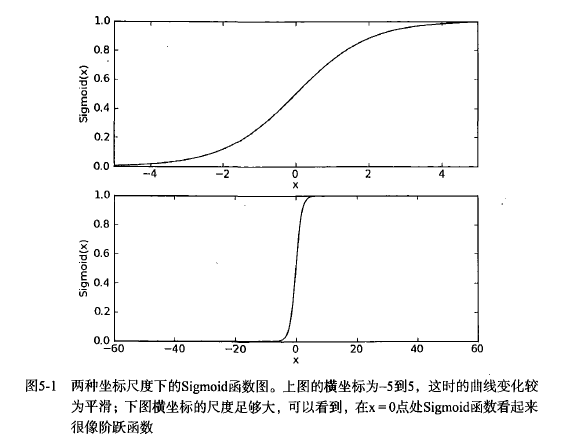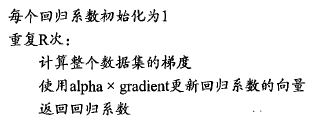from numpy import *

def sigmoid(inX):
return 1.0/(1+exp(-inX))

"""
逻辑斯谛回归梯度上升优化算法
:param dataMatIn:输入X矩阵（100*3的矩阵，每一行代表一个实例，每列分别是X0 X1 X2）
:param classLabels: 输出Y矩阵（类别标签组成的向量）
:return:权值向量
"""
dataMatrix = mat(dataMatIn)             #转换为 NumPy 矩阵数据类型
labelMat = mat(classLabels).transpose() #转换为 NumPy 矩阵数据类型
m,n = shape(dataMatrix)                 #矩阵大小
alpha = 0.001                           #步长
maxCycles = 500
weights = ones((n,1))
for k in range(maxCycles):              #最大迭代次数
h = sigmoid(dataMatrix*weights)     #矩阵内积
error = (labelMat - h)              #向量减法
weights += alpha * dataMatrix.transpose() * error  #矩阵内积
return weights

dataArr, labelMat = loadDataSet()
plotBestFit(weights)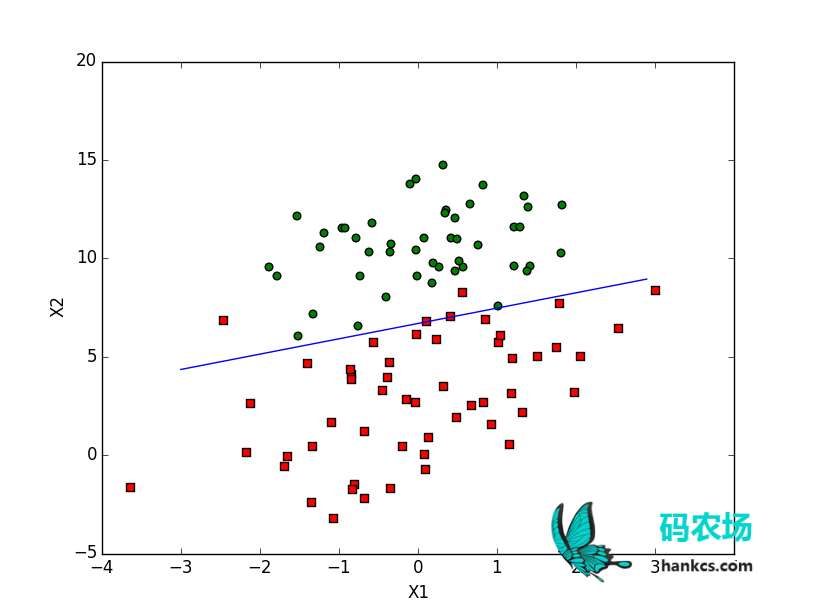# -*- coding:utf-8 -*-
# Author：hankcs
# Date: 2015/2/4 15:01
from matplotlib import pyplot as plt
from matplotlib import animation
from numpy import *

"""
加载数据集

:return:输入向量矩阵和输出向量
"""
dataMat = []; labelMat = []
fr = open('testSet.txt')
lineArr = line.strip().split()
dataMat.append([1.0, float(lineArr), float(lineArr)]) #X0设为1.0，构成拓充后的输入向量
labelMat.append(int(lineArr))
return dataMat,labelMat

def sigmoid(inX):
return 1.0/(1+exp(-inX))

"""
逻辑斯谛回归梯度上升优化算法
:param dataMatIn:输入X矩阵（100*3的矩阵，每一行代表一个实例，每列分别是X0 X1 X2）
:param classLabels: 输出Y矩阵（类别标签组成的向量）
:return:权值向量
"""
dataMatrix = mat(dataMatIn)             #转换为 NumPy 矩阵数据类型
labelMat = mat(classLabels).transpose() #转换为 NumPy 矩阵数据类型
m,n = shape(dataMatrix)                 #矩阵大小
alpha = 0.001                           #步长
maxCycles = 500
weights = ones((n,1))
for k in range(maxCycles):              #最大迭代次数
h = sigmoid(dataMatrix*weights)     #矩阵内积
error = (labelMat - h)              #向量减法
weights += alpha * dataMatrix.transpose() * error  #矩阵内积
history_weight.append(copy(weights))
return weights

history_weight = []
fig = plt.figure()
currentAxis = plt.gca()
line, = ax.plot([], [], 'b', lw=2)

def draw_line(weights):
x = arange(-5.0, 5.0, 0.1)
y = (-weights-weights*x)/weights   #令w0*x0 + w1*x1 + w2*x2 = 0，其中x0=1，解出x1和x2的关系
line.set_data(x, y)
return line,

# initialization function: plot the background of each frame
def init():
dataArr = array(dataMat)
n = shape(dataArr)
xcord1 = []; ycord1 = []
xcord2 = []; ycord2 = []
for i in range(n):
if int(labelMat[i])== 1:
xcord1.append(dataArr[i,1]); ycord1.append(dataArr[i,2])
else:
xcord2.append(dataArr[i,1]); ycord2.append(dataArr[i,2])
ax.scatter(xcord1, ycord1, s=30, c='red', marker='s')
ax.scatter(xcord2, ycord2, s=30, c='green')
plt.xlabel('X1'); plt.ylabel('X2');

return draw_line(zeros((n,1)))

# animation function.  this is called sequentially
def animate(i):
return draw_line(history_weight[i])

# call the animator.  blit=true means only re-draw the parts that have changed.
anim = animation.FuncAnimation(fig, animate, init_func=init, frames=len(history_weight), interval=10, repeat=False,
blit=True)
plt.show()
anim.save('gradAscent.gif', fps=2, writer='imagemagick')### 随机梯度上升算法

#### 随机梯度上升算法的伪代码：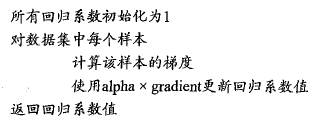#### Python实现：

def stocGradAscent0(dataMatrix, classLabels, history_weight):
"""
随机梯度上升算法
:param dataMatIn:输入X矩阵（100*3的矩阵，每一行代表一个实例，每列分别是X0 X1 X2）
:param classLabels: 输出Y矩阵（类别标签组成的向量）
:return:权值向量
"""
dataMatrix = array(dataMatrix)
m,n = shape(dataMatrix)
alpha = 0.01
weights = ones(n)                               #初始化为单位矩阵
for i in range(m):
h = sigmoid(sum(dataMatrix[i]*weights))     #挑选（伪随机）第i个实例来更新权值向量
error = classLabels[i] - h
weights = weights + dataMatrix[i] * alpha * error
history_weight.append(copy(weights))
return weights

#### 可视化：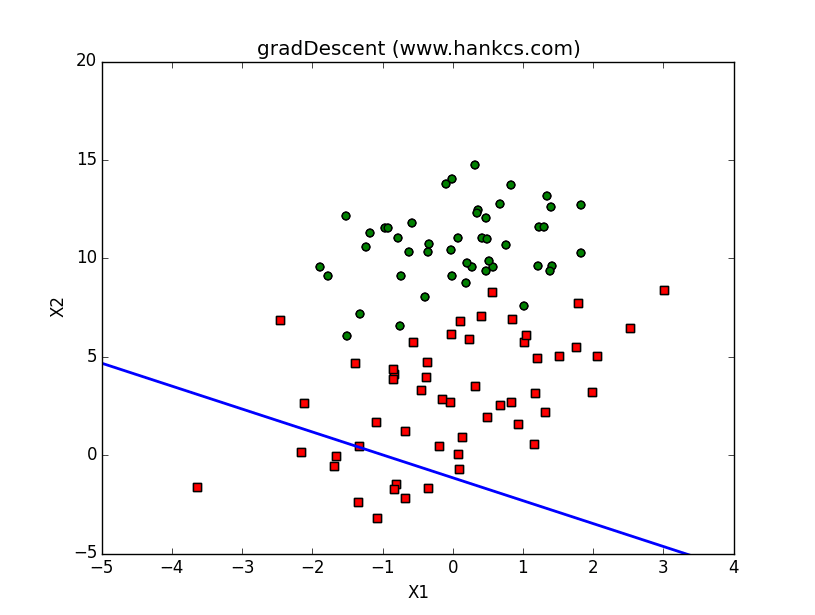### 改进的随机梯度上升算法

#### Python代码：

def stocGradAscent1(dataMatrix, classLabels, numIter=150):
"""
改进的随机梯度上升算法
:param dataMatIn:输入X矩阵（100*3的矩阵，每一行代表一个实例，每列分别是X0 X1 X2）
:param classLabels: 输出Y矩阵（类别标签组成的向量）
:param numIter: 迭代次数
:return:
"""
dataMatrix = array(dataMatrix)
m,n = shape(dataMatrix)
weights = ones(n)                                           #初始化为单位矩阵
for j in range(numIter):
dataIndex = range(m)
for i in range(m):
alpha = 4/(1.0+j+i)+0.0001                          #步长递减，但是由于常数存在，所以不会变成0
randIndex = int(random.uniform(0,len(dataIndex)))   #总算是随机了
h = sigmoid(sum(dataMatrix[randIndex]*weights))
error = classLabels[randIndex] - h
weights = weights + alpha * error * dataMatrix[randIndex]
del(dataIndex[randIndex])                           #删除这个样本，以后就不会选到了
return weights

#### 可视化：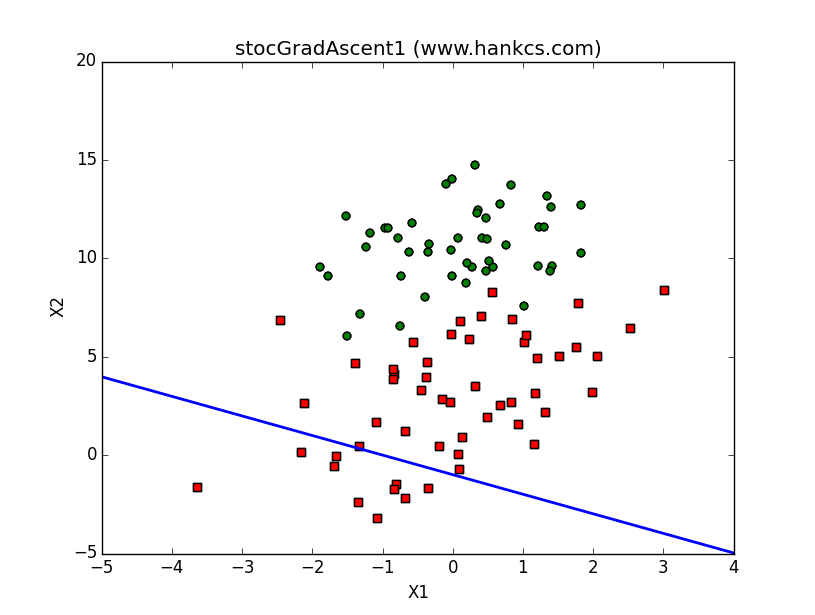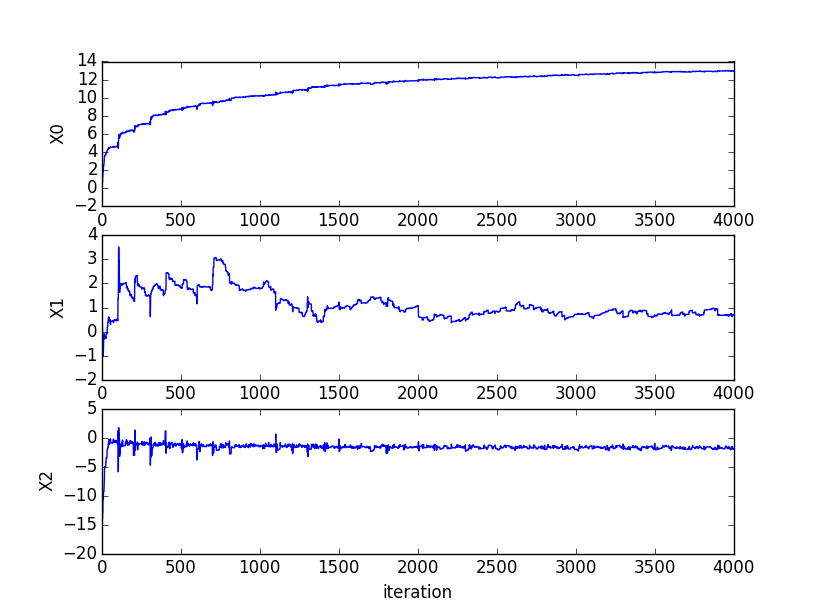## 最大熵模型

### 最大熵原理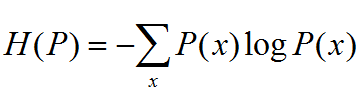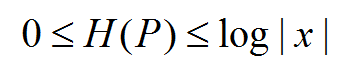|X|为X取值个数，仅当X均匀分布时，右等号成立，熵最大。

6.1 随机变量X取值{A,B,C,D,E},要估计各值的概率P(A)，P(B)…

P(A)+P(B)=3/10 -> P(A)=P(B)=3/20

P(A)+P(B)+P(C)+P(D)+P(E)=1 -> P(C)=P(D)=P(E)=7/30

### 最大熵模型的定义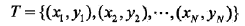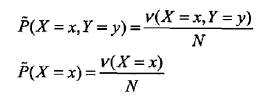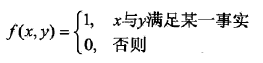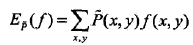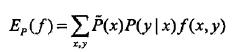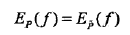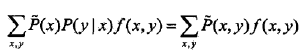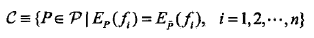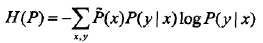### 最大熵模型的学习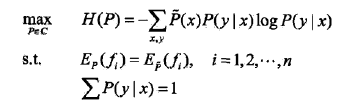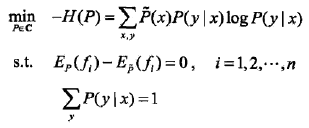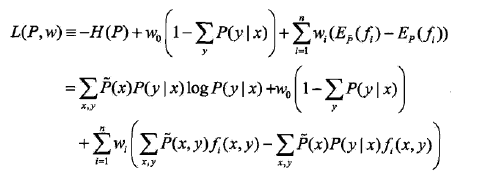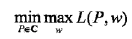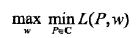《统计学习方法》介绍说由于拉格朗日函数是P的凸函数，所以可以做这种等价。这样，就可以通过求解对偶问题来求解原始问题了。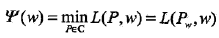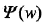称为对偶函数，同时，将其解记作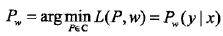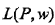对P的偏导数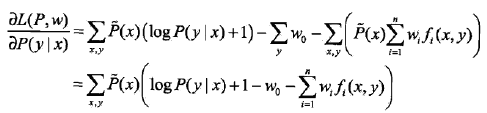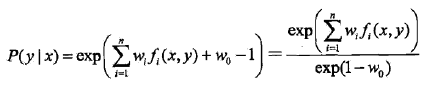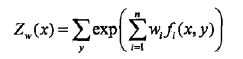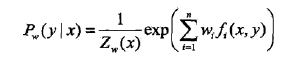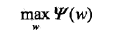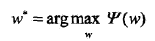### 极大似然估计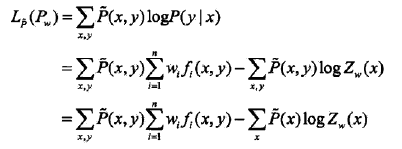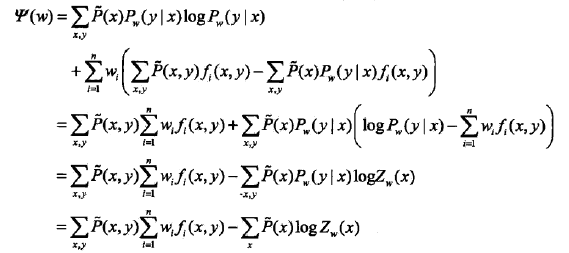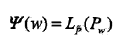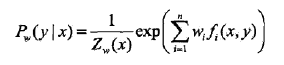## 模型学习的最优化算法

### Generalized Iterative Scaling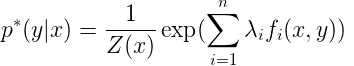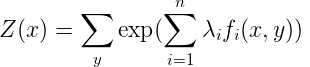GIS的算法流程如下：

1. 初始化所有λi 为任意值，一般可以设置为0，即：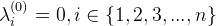2.  重复下面的权值更新直至收敛：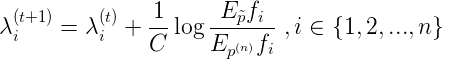# -*- coding:utf-8 -*-
# Filename: maxent.py
# Author：hankcs
# Date: 2015/10/2 23:23
import sys
import math
from collections import defaultdict

class MaxEnt:
def __init__(self):
self._samples = [] 	    #样本集, 元素是[y,x1,x2,...,xn]的元组
self._Y = set([])       #标签集合,相当于去重之后的y
self._numXY = defaultdict(int)  #Key是(xi,yi)对，Value是count(xi,yi)
self._N = 0		    #样本数量
self._n = 0		    #特征对(xi,yi)总数量
self._xyID = {}	    #对(x,y)对做的顺序编号(ID), Key是(xi,yi)对,Value是ID
self._C = 0		    #样本最大的特征数量,用于求参数时的迭代，见IIS原理说明
self._ep_ = []		#样本分布的特征期望值
self._ep = []		#模型分布的特征期望值
self._w = []		#对应n个特征的权值
self._lastw = []	#上一轮迭代的权值
self._EPS = 0.01	#判断是否收敛的阈值
for line in open(filename, "r"):
sample = line.strip().split("\t")
if len(sample) < 2: #至少：标签+一个特征
continue
y = sample
X = sample[1:]
self._samples.append(sample) #labe + features
for x in set(X):    #set给X去重
self._numXY[(x, y)] += 1
def _initparams(self):
self._N = len(self._samples)
self._n = len(self._numXY)
self._C = max([len(sample) - 1 for sample in self._samples])
self._w = [0.0] * self._n
self._lastw = self._w[:]
self._sample_ep()
def _convergence(self):
for w, lw in zip(self._w, self._lastw):
if math.fabs(w - lw) >= self._EPS:
return False
return True
def _sample_ep(self):
self._ep_ = [0.0] * self._n
#计算方法参见公式(20)
for i, xy in enumerate(self._numXY):
self._ep_[i] = self._numXY[xy] * 1.0 / self._N
self._xyID[xy] = i
def _zx(self, X):
#calculate Z(X), 计算方法参见公式(15)
ZX = 0.0
for y in self._Y:
sum = 0.0
for x in X:
if (x, y) in self._numXY:
sum += self._w[self._xyID[(x, y)]]
ZX += math.exp(sum)
return ZX
def _pyx(self, X):
#calculate p(y|x), 计算方法参见公式(22)
ZX = self._zx(X)
results = []
for y in self._Y:
sum = 0.0
for x in X:
if (x, y) in self._numXY: #这个判断相当于指示函数的作用
sum += 	self._w[self._xyID[(x, y)]]
pyx = 1.0 / ZX * math.exp(sum)
results.append((y, pyx))
return results
def _model_ep(self):
self._ep = [0.0] * self._n
#参见公式(21)
for sample in self._samples:
X = sample[1:]
pyx = self._pyx(X)
for y, p in pyx:
for x in X:
if (x, y) in self._numXY:
self._ep[self._xyID[(x, y)]] += p * 1.0 / self._N
def train(self, maxiter = 1000):
self._initparams()
for i in range(0, maxiter):
print "Iter:%d..."%i
self._lastw = self._w[:] #保存上一轮权值
self._model_ep()
#更新每个特征的权值
for i, w in enumerate(self._w):
#参考公式(19)
self._w[i] += 1.0 / self._C * math.log(self._ep_[i] / self._ep[i])
print self._w
#检查是否收敛
if self._convergence():
break
def predict(self, input):
X = input.strip().split("\t")
prob = self._pyx(X)
return prob

if __name__ == "__main__":
maxent = MaxEnt()
maxent.train()
print maxent.predict("sunny\thot\thigh\tFALSE")
print maxent.predict("overcast\thot\thigh\tFALSE")
print maxent.predict("sunny\tcool\thigh\tTRUE")
sys.exit(0)

### 改进的迭代尺度法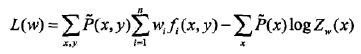IIS的思路是，假设最大熵模型当前的参数向量是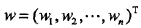，我们希望找到一个新的参数向量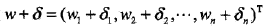，使得模型的对数似然函数值增大。如果能有这样一种参数向量更新的方法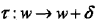，那么就可以重复使用这一方法，直至找到对数似然函数的最大值。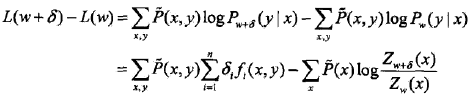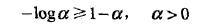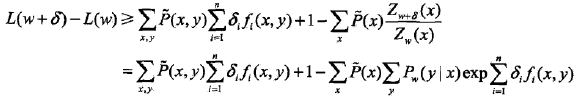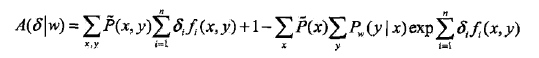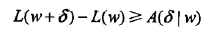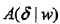是对数似然函数改变量的一个下界。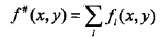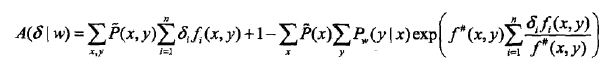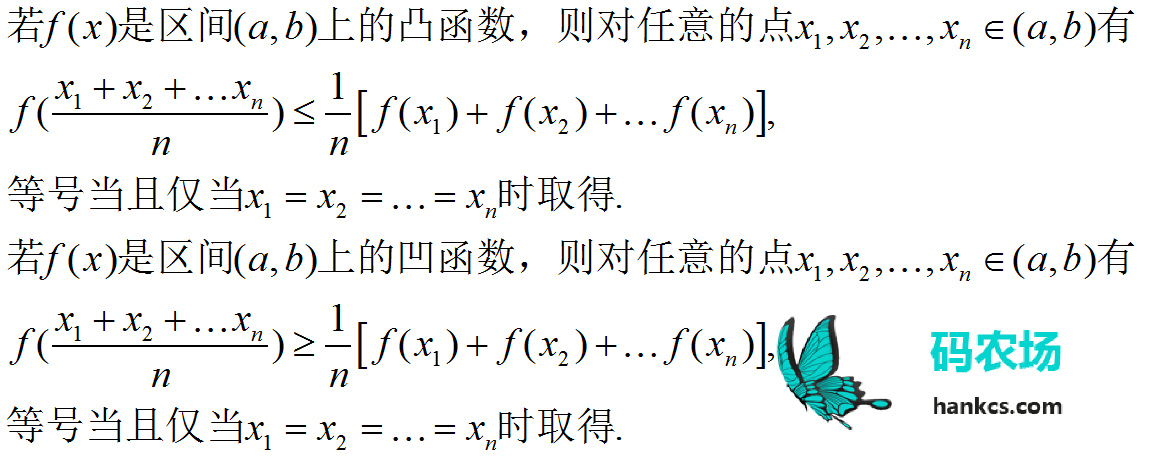，得到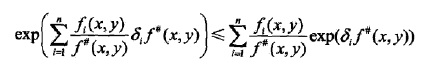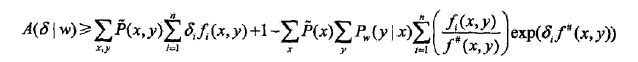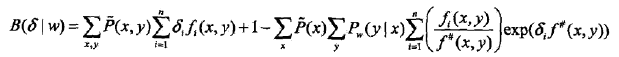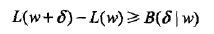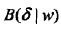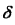的偏导数：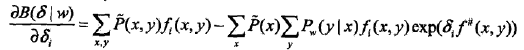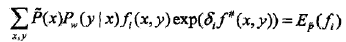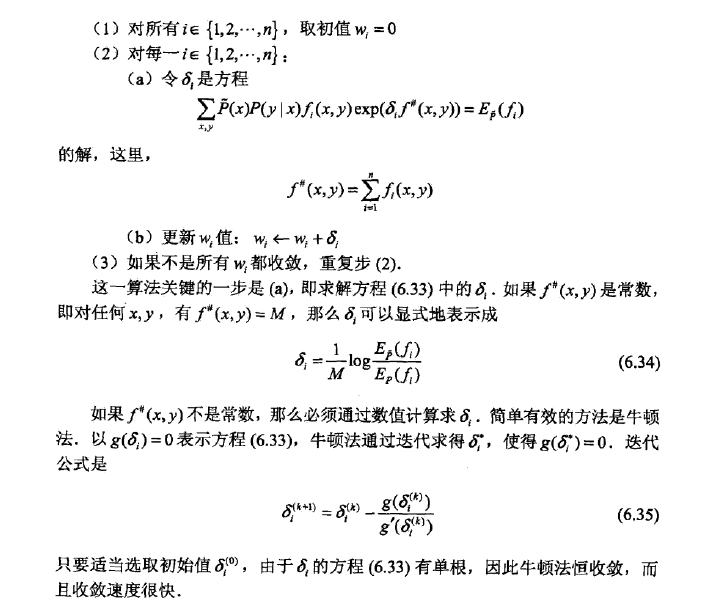#### 最大熵IIS训练算法的Java实现

Fork自 https://github.com/tpeng/maxent ，由于我已经有一个repository叫maxent了（GIS算法：https://github.com/hankcs/MaxEnt ），所以新建了这个maxent_iis，添加了中文注解，修复了数据集加载的bug，特此说明。

## Reference

《统计学习方法》

《机器学习实战》

http://www.fuqingchuan.com/2015/03/714.html

### 评论 8

1. #5

博主好，我想问下最大熵模型的特征函数是自己人工设计的吗？如果不是，那是如何实现自动化的？我在博文中没有看到说如何进行特征提取的过程

小李子5年前 (2018-04-05)回复
2. #4

博主你好。关于开篇中心对称那边应该是，F(u-x)-1/2=-F(u+x)+1/2。您再看看是否正确？

苏大天行健6年前 (2017-07-10)回复
• 感谢指正，原著写错了，我没注意到

hankcs6年前 (2017-07-10)回复
3. #3

randIndex = int(random.uniform(0,len(dataIndex))) #总算是随机了
这里应该改为randIndex = dataIndex[int(random.uniform(0,len(dataIndex)))]

葫芦僧6年前 (2017-05-20)回复
4. #2

楼主，最大熵是对偶函数的极小化等价于释然函数的极大化吧？估计你笔误，有点想问楼主，现在有没有对深度学习在NLP的应用方面的博客啊？支持楼主搞个专题，一起学习下

man7年前 (2016-04-19)回复
5. #1

Hi，楼主，很厉害的，写的很详细， 但是有一点有个疑问：
del(dataIndex[randIndex]) #删除这个样本，以后就不会选到了
这个并不是删除样本吧， 应该是随机选取样本，重复的也可以，只要重复m次即可，不知道理解的对不对

蔡军7年前 (2016-01-18)回复
• logistic回归的改进的随机梯度上升算法函数stocGradAscent1中的第16行有误 randIndex = int(random.uniform(0,len(dataIndex))) #总算是随机了 这里应该改为randIndex = dataIndex[int(random.uniform(0,len(dataIndex)))]

葫芦僧6年前 (2017-05-20)回复